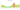# дин.

## Serbian Dinar

Serbia uses the dinar as its national currency. There are 100 paragraphs in one dinar. In Serbia, the dinar was originally used in the Middle Ages; the year of its first usage was 1214.

#### how has the value of the currency changed in the last year?## USD 1 = RSD 106.48

How much is10 US dollars worth inSerbian dinars?
At the current exchange rate, 10 US dollars is worth 1,064.84 Serbian dinars
How much is50 US dollars worth inSerbian dinars?
At the current exchange rate, 50 US dollars is worth 5,324.22 Serbian dinars
How much is100 US dollars worth inSerbian dinars?
At the current exchange rate, 100 US dollars is worth 10,648.45 Serbian dinars
How much is500 US dollars worth inSerbian dinars?
At the current exchange rate, 500 US dollars is worth 53,242.24 Serbian dinars
How much is2,000 US dollars worth inSerbian dinars?
At the current exchange rate, 2,000 US dollars is worth 212,968.94 Serbian dinars
How much is10 US dollars worth inSerbian dinars?
At the current exchange rate, 10 US dollars is worth 1,064.84 Serbian dinars
How much is50 US dollars worth inSerbian dinars?
At the current exchange rate, 50 US dollars is worth 5,324.22 Serbian dinars
How much is100 US dollars worth inSerbian dinars?
At the current exchange rate, 100 US dollars is worth 10,648.45 Serbian dinars
How much is500 US dollars worth inSerbian dinars?
At the current exchange rate, 500 US dollars is worth 53,242.24 Serbian dinars
How much is2,000 US dollars worth inSerbian dinars?
At the current exchange rate, 2,000 US dollars is worth 212,968.94 Serbian dinars